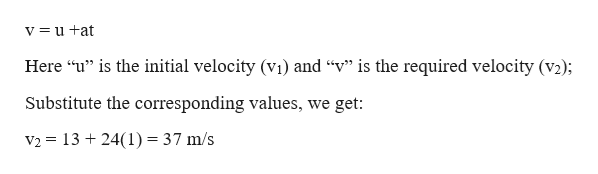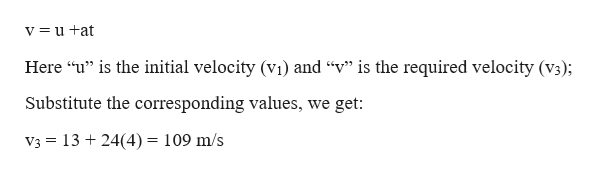# A parcel of air moving in a straight tube with a constant acceleration of 24.00 m/s2 has a velocity of 13.0 m/s at 10:05:00 a.m. (a) What is its velocity at 10:05:01 a.m.? (b) At 10:05:04 a.m.? (c) At 10:04:59 a.m.? (d) Describe the shape of a graph of velocity versus time for this parcel of air. (e) Argue for or against the following statement: “Knowing the single value of an object’s constant acceleration is like knowing a whole list of values for its velocity.”

Question
A parcel of air moving in a straight tube with a constant acceleration of 24.00 m/s2 has a velocity of 13.0 m/s at 10:05:00 a.m. (a) What is its velocity at 10:05:01 a.m.? (b) At 10:05:04 a.m.? (c) At 10:04:59 a.m.? (d) Describe the shape of a graph of velocity versus time for this parcel of air. (e) Argue for or against the following statement: “Knowing the single value of an object’s constant acceleration is like knowing a whole list of values for its velocity.”
check_circle

Step 1

Given information:

The acceleration of the air parcel of air (a) = 24 m/s2

The velocity of the parcel at 10:05:00 am (v1) = 13 m/s

Step 2

The velocity of the parcel at 10:05:01am (v2) i.e. one sec after the given velocity:

Consider the first equation of motion:help_outlineImage Transcriptionclosev=u +at Here "u" is the initial velocity (vi) and "v" is the required velocity (v2); Substitute the corresponding values, we get: V2 1324(1) = 37 m/s fullscreen
Step 3

The velocity of the parcel at 10:05:04am (v3) i.e. 4 sec after the give...help_outlineImage Transcriptionclosev=u +at Here "u" is the initial velocity (vi) and "v" is the required velocity (v3); Substitute the corresponding values, we get: V3 1324(4) = 109 m/s fullscreen

### Want to see the full answer?

See Solution

#### Want to see this answer and more?

Solutions are written by subject experts who are available 24/7. Questions are typically answered within 1 hour.*

See Solution
*Response times may vary by subject and question.
Tagged in

### Kinematics